﻿ 数字图像处理技术在金属丝弹性模量测量实验中的应用 Application of Digital Image Processing Technology in Measuring Elastic Modulus of Wire

Applied Physics
Vol. 09  No. 06 ( 2019 ), Article ID: 30801 , 5 pages
10.12677/APP.2018.96035

Application of Digital Image Processing Technology in Measuring Elastic Modulus of Wire

Wenqin Chang, Jie Zhang, Qiong Zhang, Jingfeng Wang*

Inner Mongolia University of Technology, Hohhot Inner Mongolia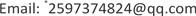Received: May 29th, 2019; accepted: Jun. 10th, 2019; published: Jun. 17th, 2019ABSTRACT

Elastic modulus is a physical quantity which describes the resistance of solid materials to deformation. Based on the traditional stretching method, and combined with the popular image processing technology, by using MATLAB data processing, this experiment designs and implements a digital image processing based on the micro-displacement measurement of elastic modulus measurement system.

Keywords:Modulus of Elasticity1. 引言

2. 实验方案

$E=\frac{F/A}{n/L}=\frac{4\text{mgL}}{{\text{πd}}^{2}n}$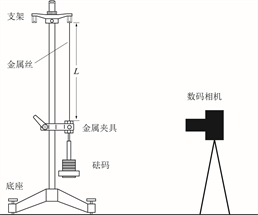Figure 1. Schematic diagram of the experimental device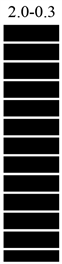Figure 2. Schematic diagram of markers

3. 数据处理

$F=\left[\begin{array}{cccc}f\left(0,0\right)& f\left(0,1\right)& \dots & f\left(0,n-1\right)\\ f\left(1,0\right)& f\left(1,1\right)& \dots & f\left(1,n-1\right)\\ ⋮& ⋮& ⋮& ⋮\\ f\left(m-1,0\right)& f\left(m-1,1\right)& \dots & f\left(m-1,n-1\right)\end{array}\right]$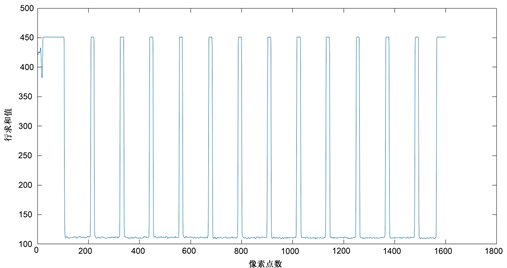Figure 3. Schematic diagram of the relationship between matrix row values and pixel points

$S=\frac{2mm}{size\left(一个黑色部分的宽所占像素点数\right)}$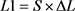Table 1. Wire extension pixel count () and actual elongation (L1)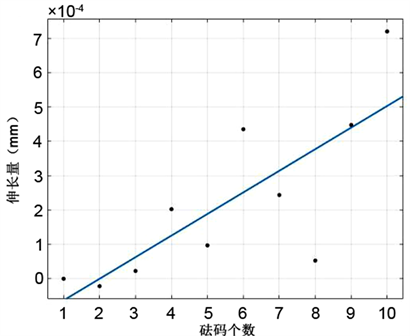Figure 4. Schematic diagram of data fitting results

4. 结语

Application of Digital Image Processing Technology in Measuring Elastic Modulus of Wire[J]. 应用物理, 2019, 09(06): 295-299. https://doi.org/10.12677/APP.2018.96035

1. 1. 杨欣欣, 应黎坪, 彭一江. 基于数字图像处理技术的再生混凝土数值模拟[J]. 河北工业科技, 2018(5): 1-6.

2. 2. 单宝华, 霍晓洋, 刘洋. 基于MATLAB的DIC方法位移测量教学实验研究[J]. 实验室科学, 2016, 19(2): 43-45+49.

3. NOTES

*通讯作者。